1. Kindly refer the question below:

Two galaxies with an angular separation at the observer of   5.0 × 10^(−4)  radians are observed with a radio telescope. Both galaxies emit radio waves of wavelength 2.5×10^(−2) m.

The images of the galaxies are just resolved by the telescope. The diameter of the circular collecting dish of the telescope is

2. A diffraction grating is used to observe light of wavelength 400 nm. The light illuminates 100 slits of the grating. What is the minimum wavelength difference that can be resolved when the second order of diffraction is viewed?
3. Kindly refer the question below:

A mass oscillates with simple harmonic motion (SHM) of amplitude xo. Its total energy is 16 J.

What is the kinetic energy of the mass when its displacement is x_0/2?

4. Monochromatic light is incident on two identical slits to produce an interference pattern on a screen. One slit is then covered so that no light emerges from it. What is the change to the pattern observed on the screen?
5. Two lines X and Y in the emission spectrum of hydrogen gas are measured by an observer stationary with respect to the gas sample.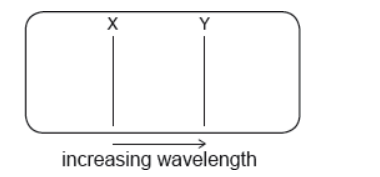The emission spectrum is then measured by an observer moving away from the gas sample.

What are the correct shifts X* and Y* for spectral lines X and Y?

6. Kindly refer the question below:

Light of wavelength lambda is incident normally on a diffraction grating that has a slit separation of . What is the greatest number of maxima that can be observed using this arrangement?

7. Which of the following wave phenomena is associated with blood flow measurements?
8. Monochromatic light of wavelength λ in air is incident normally on a thin film of refractive index n. The film is surrounded by air. The intensity of the reflected light is a minimum. What is a possible thickness of the film?
9. Monochromatic light is incident on 4 rectangular, parallel slits.

The first principal maximum is observed at an angle θ to the direction of the incident light. The number of slits is increased to 8 each having the same width and spacing as the first 4.

Three statements about the first principal maximum with 8 slits are

I.     the angle at which it is observed is greater than θ

II.     its intensity increases

III.     its width decreases.

Which statements are correct?

10. A simple pendulum bob oscillates as shown.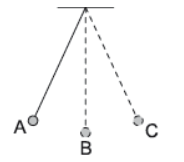At which position is the resultant force on the pendulum bob zero?

11. A pendulum oscillating near the surface of the Earth swings with a time period T. What is the time period of the same pendulum near the surface of the planet Mercury where the gravitational field strength is 0.4g?
12. A spring loaded with mass m oscillates with simple harmonic motion. The amplitude of the motion is A and the spring has total energy E. What is the total energy of the spring when the mass is increased to 3m and the amplitude is increased to 2A?
13. A beam of coherent light is incident on a single slit of width b. After passing through the slit, the light is incident on a screen at a distance D from the slit.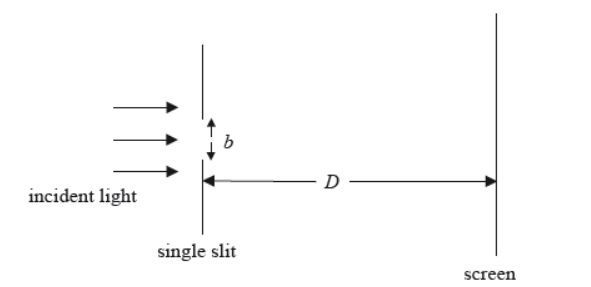Which of the following changes, carried out separately, in respect of and will result in an increase in width of the first diffraction maximum formed on the screen?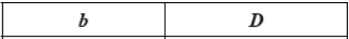14. A parallel beam of coherent light of wavelength λ is incident on a rectangular slit of width d. After passing through the slit the light is incident on a screen a distance D from the slit where D is much greater than d. What is the width of the central maximum of the diffraction pattern as measured on the screen?
15. A single-slit diffraction experiment is performed using light of different colours. The width of the central peak in the diffraction pattern is measured for each colour. What is the order of the colours that corresponds to increasing widths of the central peak?
16. A beam of monochromatic light is incident on a single slit and a diffraction pattern forms on the screen.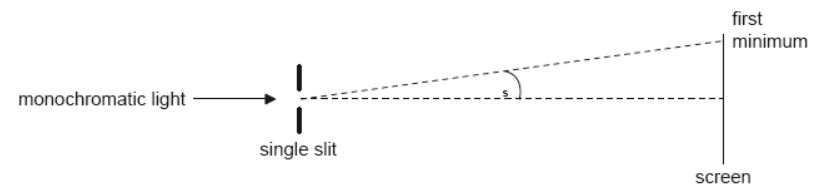What change will increase θs?

17. Green light is emitted by two point sources. The light passes through a narrow slit and is received by an observer. The images of the two sources just fail to be resolved. Which change allows for the images to be resolved?
18. A mass at the end of a vertical spring and a simple pendulum perform oscillations on Earth that are simple harmonic with time period T. Both the pendulum and the mass-spring system are taken to the Moon. The acceleration of free fall on the Moon is smaller than that on Earth. What is correct about the time periods of the pendulum and the mass-spring system on the Moon?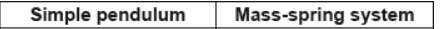19. A train travelling in a straight line emits a sound of constant frequency f. An observer at rest very close to the path of the train detects a sound of continuously decreasing frequency. The train is
20. Kindly refer the question below:

A particle is oscillating with simple harmonic motion (shm) of amplitude x0 and maximum kinetic energy Ek. What is the potential energy of the system when the particle is a distance 0.20x0 from its maximum displacement?

21. Which of the following experiments provides evidence for the existence of matter waves?
22. A transparent liquid forms a parallel-sided thin film in air. The diagram shows a ray I incident on the upper air–film boundary at normal incidence (the rays are shown at an angle to the normal for clarity).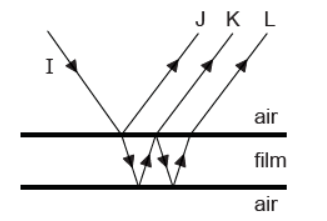Reflections from the top and bottom surfaces of the film result in three rays J, K and L. Which of the rays has undergone a phase change of  rad?

23. A beam of monochromatic light is incident on a diffraction grating of N lines per unit length.

The angle between the first orders is θ1.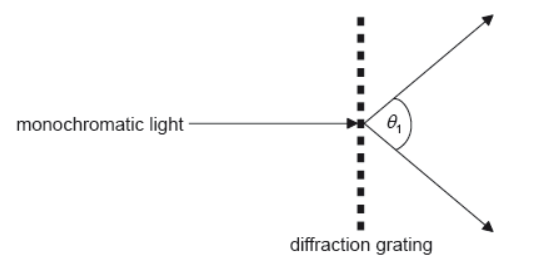What is the wavelength of the light?

24. Two waves meet at a point.

The waves have a path difference of λ/4. The phase difference between the waves is

25. A simple pendulum has mass M and length l. The period of oscillation of the pendulum is T. What is the period of oscillation of a pendulum with mass 4M and length 0.25l?
26. A source of sound approaches a stationary observer.

The speed of the emitted sound and its wavelength, measured at the source, are nu and λ respectively. Which of the following is the wave speed and the wavelength, as measured by the stationary observer?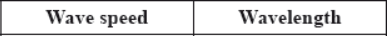27. A stationary source of sound emits sound of frequency f.

A moving observer measures the sound as having the frequency f ′. The observer is moving directly away from the source at a speed that is 30% of the speed of sound in air.

Which of the following gives the correct value for (f′)/f?

28. An object to be viewed by a microscope is irradiated with blue light. The reason for using blue light rather than light of a longer wavelength is to increase
29. The images of two sources are just resolved. Which of the following is a correct statement of the Rayleigh criterion for this situation?
30. Light of wavelength λ is emitted by two point sources.

The light passes through a circular aperture of diameter b and is received by an observer. The angular separation of the sources from the observer’s position is θ. The sources are not resolved by the observer. Which of the following mathematical relationships applies?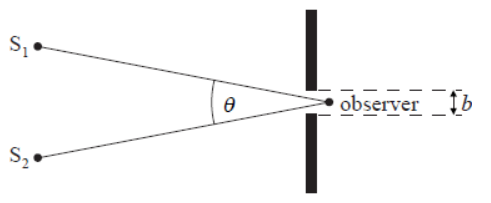31. A car horn emits sound of frequency ƒ. While the horn is sounding, the car moves in a straight line towards a stationary observer. The speed of the car is 0.10v where v is the speed of sound. What is the frequency of the sound of the horn heard by the observer?
32. A train is approaching an observer with constant speed

c/34

where c is the speed of sound in still air. The train emits sound of wavelength λ. What is the observed speed of the sound and observed wavelength as the train approaches?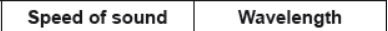33. Kindly refer the question below:

A source emits sound of wavelength and wave speed . A stationary observer hears the sound as the source moves away. What are the wavelength of the sound and the wave speed of the sound as measured by the stationary observer?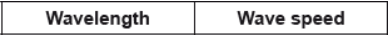34. A siren on an ambulance emits sound of frequency f.

The speed of sound in still air is v. What is the frequency of the sound observed when the ambulance travels at speed v/10 towards a stationary observer?

35. During a journey an observer travels at constant speed towards, and then goes beyond, a stationary emitter of sound.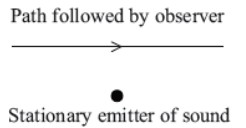The frequency of the sound as measured at the emitter is . The frequency according to the observer

36. Kindly refer the question below:

A body moves with simple harmonic motion (SHM) with period T and total energy ET. What is the total energy when the period of the motion is changed to 5T and the amplitude of the motion remains constant?

37. A sample of hydrogen on Earth emits a spectral line that is measured by an Earth observer to have wavelength 500 nm. The same spectral line is emitted by a galactic source that is moving away from Earth at speed of 0.1c. What is the wavelength of the galactic spectral line that will be measured by the Earth observer?
38. Kindly refer the question below:

Two point sources of light have an angular separation of θ, as measured by a distant observer. The light passes through a circular aperture of radius r just before reaching the observer. Which of the following conditions must be true for the two sources to be resolved?

39. A radio telescope has a circular collecting dish of diameter 5.0 m. It is used to observe two distant galaxies that are both emitting electromagnetic radiation of wavelength 20 cm. The images of the galaxies are just resolved by the telescope. What is the angle subtended by the galaxies at the telescope?
40. A source of sound moves away from an observer. The observed frequency of the sound differs from the frequency emitted by the source because the
41. Which of the following would be diffracted the most when incident on a slit of width 1 cm?
42. Blue light is incident on two narrow slits. Constructive interference takes place along the lines labelled 1 to 5.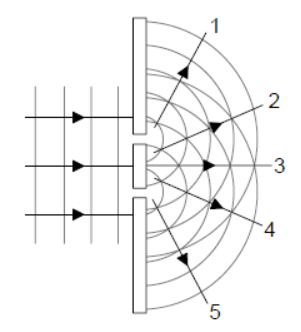The blue light is now replaced by red light. What additional change is needed so that the lines of constructive interference remain in the same angular positions?

43. Kindly refer the question below:

A train moves at constant speed whilst emitting a sound wave of frequency f0. At t=t0 the train passes through a station. Which graph shows the variation with time t of the frequency f of the sound wave as measured by an observer standing on the station platform?

44. A stationary sound source emits waves of wavelength λ and speed v. The source now moves away from a stationary observer. What are the wavelength and speed of the sound as measured by the observer?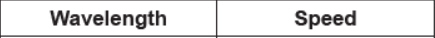45. A parallel beam of monochromatic light of wavelength λ passes through a slit of width b. After passing through the slit the light is incident on a distant screen. The angular width of the central maximum is